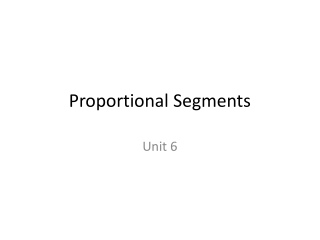DownloadDownload PresentationProportional Segments

# Proportional Segments

Télécharger la présentation## Proportional Segments

- - - - - - - - - - - - - - - - - - - - - - - - - - - E N D - - - - - - - - - - - - - - - - - - - - - - - - - - -
##### Presentation Transcript

1. Proportional Segments Unit 6

2. Corresponding Angles Alternate Interior Angles

3. Let’s go over your HW. • What is the number of the vertical angle of  3? •  2 • What is the number of the corresponding angle of  3? •  7 • What is the number of the alternate interior angle of  3? •  6 • What is the number of the same side interior angle of  3? •  5 • The number of the supplementary angle of  3? •  1 and  4 • If m 3 were 80, what would be the m  1? • 100

4. Open Note Quiz • Get out the notes that you took yesterday.

5. Copy the picture above. • What are the measurements of 1 and 2? • What are the measurements that we know? • Does z have an angle relationship with 1 or 2? • Does 110 have an angle relationship with 1 or 2? • What does z equal? (Hint: z + 110 = 180) • What are the measurements of 1 and 2? z 110

6. Copy the picture above. • What are the measurements of 1 and 2? • What are the measurements that we know? • Does x have an angle relationship with 1 or 2? • Does 2x have an angle relationship with 1 or 2? • What does x equal? (Hint: x + 2x = 180) • What are the measurements of 1 and 2? 7 x 2x

7. Write these equations. left top = right top left total right total left top__ = right top left bottom right bottom left top__ = left total__ middle line bottom line

8. HW • Algebra Applications with Angles WS • Practice with Similar Figures WS

9. Go to other PPT.